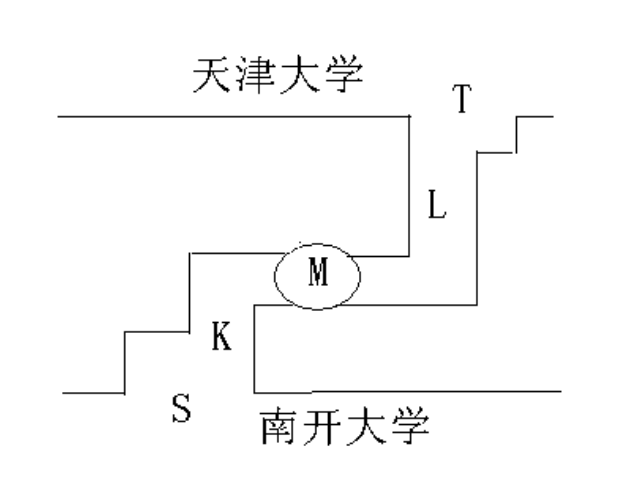有一个仓库，可以存放 A 和 B 两种产品，仓库的存储空间足够大，但要求: (1)一次只能存入一种产品(A 或 B); (2)-N < (A 产品数量-B 产品数量) < M。 其中，N 和 M 是正整数。试用“存放 A”和“存放 B”以及 P、V 操作描述产品 A 与 产品 B 的入库过程。

Semaphore Sa = M - 1;
Semaphore Sb = N - 1;
//代表还能存入的数量

Semaphore mutex = 1;

process_A() {
while(1){
P(Sa); //取一个A产品准备入库
P(mutex);
A产品入库;
// A产品入库后还能存入A产品数量-1
V(mutex);
V(Sb); //还能存入B产品数量+1
}
}

process_B() {
while(1){
P(Sb); //取一个B产品准备入库
P(mutex);
B产品入库;
// B产品入库后还能存入B产品数量-1
V(mutex);
V(Sa); //还能存入A产品数量+1
}
}


桌子上有一只盘子，最多可容纳两个水果，每次只能放入或取出一个水果。爸爸专向盘子放苹果（ apple），妈妈专向盘子中放桔子（ orange）；两个儿子专等吃盘子中的桔子，两个女儿专等吃盘子中的苹果。请用 P、 V 操作来实现爸爸、妈妈、儿子、女儿之间的同步与互斥关系。

Semaphore mutex = 1;      //互斥信号量, 其初值为1
Semaphore empty = 2;       //记录允许向盘子中放入水果的个数，初值为2
Semaphore orange = 0;      //盘子中已放入的苹果的个数，初值为0
Semaphore apple = 0;      //盘子中已放入的桔子的个数，初值为0
main()
{
Cobegin
{
father                    //父亲进程
{
while (true)
{
P(empty);           //减少盘中可放入的水果数
P(mutex);           //申请向盘中取、放水果
向盘中放苹果;
V(mutex);           //允许向盘中取、放水果
V(apple);           //递增盘中的苹果数
}
}
mother                    //母亲进程
{
while (true)
{
P(empty);           //减少盘中可放入的水果数
P(mutex);           //申请向盘中取、放水果
向盘中放桔子;
V(mutex);           //允许向盘中取、放水果
V(orange);          //递增盘中的桔子数
}
}
daughteri（i=1,2）      //两女儿进程
{
while (true)
{
P(apple);           //减少盘中苹果数
P(mutex);           //申请向盘中取、放水果
取盘中苹果;
V(mutex);           //允许向盘中取、放水果
V(empty);           //递增盘中可放入的水果数
}
}
sonj（j=1,2）           //两儿子进程
{
while (true)
{
P(orange);          //减少盘中桔子数
P(mutex);           //申请向盘中取、放水果
取盘中桔子;
V(mutex);           ／/允许向盘中取、放水果
V(empty);           //递增盘中可放入的水果数
}
}
}
Coend
}


有一个理发师，一把理发椅和 N 把供等候理发的顾客坐的椅子。如果没有顾客，则理发师便在理发师椅子上睡觉；当一个顾客到来时，必须唤醒理发师进行理发；如果理发师正在理发时又有顾客来到，则如果有空椅子可坐，他就坐下来等，如果没有空椅子，他就离开。为理发师和顾客各编一段程序（伪代码）描述他们的行为，要求不能带有竞争条件。

Semaphore mutex = 1;    //互斥信号量，初值为1.
Semaphore  Wait = 0;     //等待服务的顾客数
Semaphore  barbers= 0;    //等待顾客的理发师数
Int custNum = 0;    //等待的顾客(还没理发的)

Costumer()
{
while(true)
{
P(mutex);            //申请理发
if(custNum>0)
{
if(custNum<N)   //若等待人数小于N
{
V(mutex);     //释放进程等待
CustNum++；     //增加等待人数
}
else            //若等待人数超过N
{
V(mutex);   //释放进程等待
离开；
}
}
else                //若目前无人等待
{
V(mutex);        //释放进程等待
V(barbers)；     //如果必要的话，唤醒理发师
理发；
离开；
P(mutex);        //要求进程等待
custNum--；        //顾客人数减1
V(mutex);       //释放进程等待
V(wait);        //等待人数减1
}
}
}

Barber()
{
while(true)
{
P(mutex);            //要求进程等待
if(custNum ==0)    //目前无顾客
{
V(mutex);        //释放进程等待
P(barbers);        //理发师睡觉
}
else
{
V(mutex);        //释放进程等待
理发;
}
}
}


吸烟者问题。三个吸烟者在一间房间内，还有一个香烟供应者。为了制造并抽掉香烟，每个吸烟者需要三样东西：烟草、纸和火柴。供应者有丰富的货物提供。三个吸烟者中，第一个有自己的烟草，第二个有自己的纸，第三个有自己的火柴。供应者将两样东西放在桌子上，允许一个吸烟者进行对健康不利的吸烟。当吸烟者完成吸烟后唤醒供应者，供应者再放两样东西（随机地）在桌面上，然后唤醒另一个吸烟者。试为吸烟者和供应者编写程序解决问题。

Semaphore S = 1;                //供应者
Semaphore S1,S2,S3;                //三个吸烟者
S1 = S2 = S3 = 0;
bool flag1,flag2,fiag3;            //三种吸烟原料
fiag1=flag2=flag3=true;

Apply()                            //供应者
{
While(true)
{
P(S);
取两样香烟原料放桌上，由flagi标记；
if (flag2 && flag3) //供纸和火柴
{
V(S1);          //唤醒吸烟者一
}
else if(flag1 && fiag3) //供烟草和火柴
{
V(S2);                //唤醒吸烟者二
}
else                      //供烟草和纸
{
V(S3);                //唤醒吸烟者三
}
}
}

Smoker1()                         //吸烟者一
{
While(true)
{
P(S1);
取原料；
做香烟；
V(S);                    //唤醒供应者
吸香烟；
}
}

smoker2()                        //吸烟者二
{
While(true)
{
P(S2);
取原料；
做香烟；
V(S);                    //唤醒供应者
吸香烟;
}
}

Smoker3()                        //吸烟者三
{
While(true)
{
P(S3);
取原料；
做香烟；
V(S);                    //唤醒供应者
吸香烟;
}
}


面包师问题。面包师有很多面包和蛋糕，由 n 个销售人员销售。每个顾客进店后先取一个号，并且等着叫号。当一个销售人员空闲下来，就叫下一个号。请分别编写销售人员和顾客进程的程序。

Semaphore buyer= 0;                //顾客人数
Semaphore seller = n;            //销售人员数
Semaphore mutex_s = 1;            //用于销售人员的互斥信号量
Semaphore mutex_b = 1;            //用于顾客的互斥信号量
int count_s = 0;                //记录取号的值
int count_b = 0;                //记录叫号的值

{
进店；
P(mutex_b);          //取号
count_b++;
V(mutex_b);
P(seller);            //等待叫号
买面包；
离开
}

void Sell()
{
while(true)
{
P(mutex_s);   //叫号
count_s++;
叫编号为count_s的顾客；
V(mutex_s);
V(seller)；
}
}


桌上有一空盘，运行存放一只水果，爸爸可向盘中放苹果，也可放桔子，儿子专等吃盘中的桔子，女儿专等吃盘中的苹果。规定当盘中空时一次只能放一个水果供吃者取用，用 P,V 原语实现爸爸儿子和女儿 3 个并发进程的同步。

	Semaphore S = 1;      //S 表示盘子是否为空；
Semaphore Sa = 0;        //Sa 表示盘中是否有苹果；
Semaphore Sb = 0;    //Sb 表示盘中是否有桔子；

Father()            //父亲进程
{
while(TRUE)
{
P(S);
将水果放入盘中;
if (放入的是桔子)
V(Sb);
else
V(Sa);
}
}

Son()                  //儿子进程
{
while(TRUE)
{
P(Sb);
从盘中取出桔子；
V(S);
吃桔子;
}
}

Daughter()            //女儿进程
{
while(TRUE)
{
P(Sa);
从盘中取出苹果；
V(S);
吃苹果;
}
}


写者优先的读者－－写者问题。读者-写者问题为数据库访问建立了一个模型。例如,一个系统,其中有许多竞争的进程试图读写其中的数据,多个进程同时读是可以接受的,但如果一个进程正在更新数据库,则所有的其他进程都不能访问数据库，即使读操作也不行。写者优先是指当一个写者到达时，将阻止其后面的读者进入数据库，直到其离开为止。

	Semaphore Mut1, Mut2, Wmutex, Fmutex;          //互斥信号量
int Rcount, Wcount;                          //读写者人数
Mut1 = Mut2 = WMutex = Fmutex = 1;
Rcount = Wcount = 0;

Writer()                            //写者进程
{
While(true)
{
P(Mut1);
Wcount=Wcount+1；
If (Wcount==1)
{
P(Fmutex);     //如有读者，写者阻塞在此处
}
V(Mut1);
P(WMutex);
写；
V(Wmutex);
P(Mut1);
Wcount=Wcount-1;
If (Wcount==0)
{
V(Fmutex);
}
V(Mut1);
}
}

{
While(true)
{
P(Mut2);
Rcount=Rcount+1;
If (Rcount==1)
{
P(Fmutex);
}
V(Mut2);
读；
P(Mut2);
Rcount=Rcount-1;
If (Rcount==0)
{
V(Fmutex);
}
V(Mut2);
}
}


在天津大学与南开大学之间有一条弯曲的小路，这条路上每次每个方向上只允许一辆自行车通过。但其中有一个小的安全岛 M，同时允许两辆自行车停留，可供两辆自行车已从两端进入小路的情况下错车使用。如图所示。begin
t:=1;s:=1;l:=1;k:=1;
cobegin
从天大到南开的进程
begin
______(1)______
通过 L 路段;
进入安全岛 M；
______(2)______
通过 K 路段
______(3)______
end
从南开到天大的进程
begin
略，与“从天大到南开的进程”相反。
end
coend
end


解答：

(1) P(t); P(l);

(2) V(l); P(k);

(3) V(k); V(t);

三个进程 P1、 P2、 P3 互斥使用一个包含 N(N>0)个单元的缓冲区。 P1 每次用 produce()生成一个正整数并用 put()送入缓冲区某一空单元中;P2 每次用 getodd()从该缓冲区中取出一个奇数并用 countodd()统计奇数个数;P3 每次用 geteven()从该缓冲区中取出一个偶数并用 counteven()统计偶数个数。请用信号量机制实现这三个进程的同步与互斥活动,并说明所定义信号量的含义。要求用伪代码描述。

P1()
{
While(true)
{
X = produce();      //生成一个数
P(empty);     //是否有空单元格
P(mutex);    //进入临界区
Put();
if(X%2 == 0)
V(s2);   //如果是偶数，向P3发出信号
else
V(s1);   //如果是奇数，向P2发出信号
V(mutex);         //离开临界区，释放互斥信号量
}
}

P2()
{
While(true)
{
P(s1);     //收到P1发送来的信号，已产生奇数
P(mutex);         //进入临界区
getodd();
countodd():=countodd()+1;
V(mutex);
V(empty);         //离开临界区，释放互斥信号量
}
}

P3()
{
While(true)
{
P(s2)        //收到P1发送来的信号，已产生偶数
P(mutex);         //进入临界区
geteven();
counteven():=counteven()+1;
V(mutex);
V(empty);         //离开临界区，释放互斥信号量
}
}